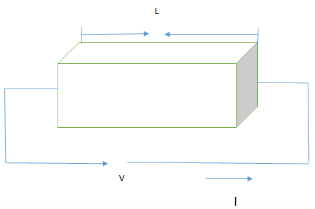Courses

# Test: Conductivity Of A Semiconductor

## 10 Questions MCQ Test Electronic Devices | Test: Conductivity Of A Semiconductor

Description
This mock test of Test: Conductivity Of A Semiconductor for Electrical Engineering (EE) helps you for every Electrical Engineering (EE) entrance exam. This contains 10 Multiple Choice Questions for Electrical Engineering (EE) Test: Conductivity Of A Semiconductor (mcq) to study with solutions a complete question bank. The solved questions answers in this Test: Conductivity Of A Semiconductor quiz give you a good mix of easy questions and tough questions. Electrical Engineering (EE) students definitely take this Test: Conductivity Of A Semiconductor exercise for a better result in the exam. You can find other Test: Conductivity Of A Semiconductor extra questions, long questions & short questions for Electrical Engineering (EE) on EduRev as well by searching above.
QUESTION: 1

### What is the SI unit of conductivity?

Solution:

The formula of the conductivity is the σ=1/ρ.
So, the unit of resistivity is Ωm.
Now, the unit of conductivity becomes the inverse of resistivity.

QUESTION: 2

### Which of the following expressions doesn’t represent the correct formula for Drift current density?

Solution:

The following formulae represent the correct expression for drift current density,
J=σE
And J=qnµE.

QUESTION: 3

### Does a semiconductor satisfy the ohm’s law?

Solution:

V=IR
J=σE
I/A=σ(V/L)
V=(L/ σA)*I=(ρL)*I/A=IR
Thus, above equation satisfies Ohm’s law.

QUESTION: 4

In which range of temperature, freeze out point begins to occur?

Solution:

At lower range of temperature, the concentration and conductivity decreases with lowering of the temperature.

QUESTION: 5

Which of the following expression represents the correct formula for the conductivity in an intrinsic material?

Solution:

Option b is the correct formula.

QUESTION: 6

What is the voltage difference if the current is 1mA and length and area is 2cm and 4cm2 respectively?(ρ=2Ωm)

Solution:

V=IR
R=ρl/(A)=2*2/4=100Ω
V=1mA*100
=0.1V.

QUESTION: 7

Is resistivity is a function of temperature?

Solution:

Resistance depends on the temperature and the resistivity depends on the resistance, so now the resistivity depends on the temperature.

QUESTION: 8

What is the electric field when the voltage applied is 5V and the length is 100cm?

Solution:

E=V/L=5/100cm=5V/m.

QUESTION: 9

Calculate the average random thermal energy at T=300K?

Solution:

Average random thermal energy=3/2*k*T=0.038eV.

QUESTION: 10In the above figure, a semiconductor having an area ‘A’ and length ‘L’ and carrying current ‘I’ applied a voltage of ‘V’ volts across it. Calculate the relation between V and A?

Solution:

Option A, satisfies the Ohm’s law which is V=IR where R=(ρl)/A.